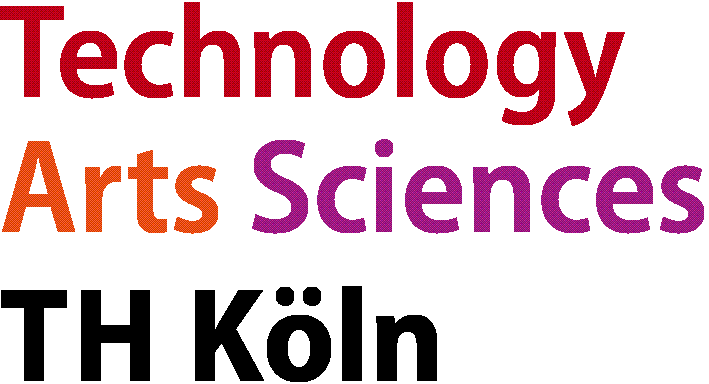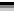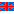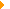Master of Eng. in Automation & ITDeutschEnglischAutomation & ITCourseModulesOptimization

# Optimization of Technical SystemsQualification aims

Students will learn, understand and be able to apply to technical processes

• practical optimization using precise mathematical and stochastic procedures

In particular, they will use "state of the art" optimization software in order to

• record and analyse new tasks and problems,
• choose suitable solution methods,
• ascertain and evaluate correct solutions.

Students can

• optimize technical systems
• implement, train and debug neural networks
• judge the importance of human-centered AI
• consider fairness, transparency, and ethics in AI

by

• understanding, applying and evaluating numerical methods and algorithms
• understanding and applying optimization theory
• understanding and applying machine learning and artificial intelligence methods and algorithms
• analyzing new tasks and problems
• choosing suitable optimization methods
• using “state of the art” optimization software and optimization algorithms
• implementing representations, image features
• applying optimization algorithms (stochastic gradient descent)
• understanding backpropagation
• choosing suitable network architectures
• analyzing generative models
• ascertaining and evaluation correct solutions
• understanding bias in data
• using tools for visualizing model states
• summarizing results in reports
• presenting results in oral presentations

to

• be able to improve the behavior of technical systems
• solve practical engineering tasks in classification and prediction
• be qualified for a professional career as automation engineer

Courses

The module consists of three courses:

Numerical Methods

Tutor

Prof. Bartz-Beielstein

Credit points

3 CP

Term

Fall

Contents

• Matrices
• Differences, Derivatives, and Boundary Conditions
• Inverses and Delta Functions
• Eigenvalues and Eigenvectors
• Positive Definite Matrices
• Numerical Linear Algebra: LU, QR, SVD
• Numerical integration of standard differential equation systems (linear, non-linear, formal procedures (Runge-Kutta etc.)
• Boundary value problems
• Differential Equations of Equilibrium
• Cubic Splines and Fourth Order Equations
• Gradient and Divergence
• Laplace's Equation
• Finite Differences and Fast Poisson Solvers
• The Finite Element Method
• Stochastic simulation
• Design and organisation of a Monte Carlo simulator

Optimization

Tutor

Prof. Bartz-Beielstein

Credit points

4 CP

Term

Fall

Contents

• Optimization criteria
• Optimization basics (calculus of variation, Euler formula, Hamilton formula, maximum principle, etc.)
• Linear Programming (LP)
• Nonlinear Programming (NLP)
• Quadratic Programming (QP)
• Integer Programming (IP)
• Direct (extrapolation-free) searching procedures (pattern search)
• Stochastic procedures (simulated annealing, evolutionary algorithms)
• Application of optimization procedures to practical problems

Machine Learning and AI

Tutor

Prof. Bartz-Beielstein

Credit points

3 CP

Term

Spring

Contents

• Image Classification: Data-driven Approach, k-Nearest Neighbor, train/val/test splits, L1/L2 distances, cross-validation
• Linear Regression, Logistic Regression, Softmax Regression
• Optimization: Stochastic Gradient Descent
• Neural Networks, Backpropagation
• Convolutional Neural Networks: Architectures, Convolution / Pooling Layers
• Understanding and Visualizing Convolutional Neural Networks

Bibliography

• Stoer, J., et.al.: Introduction to numerical analysis. ISBN 0-387-95452-X
• Kincaid, D., et.al.: Numerical analysis. ISBN 0-534-38905-8
• Gill, P.E., Murray, W., Wright, M.: Practical Optimization. Academic Press, London, 1989
• Edgar, T.F., Himmelblau, D.M.: Optimization of chemical processes. Mc Graw-Hill, 2001
• Gekeler, E.W.: Mathematical Methods for Mechanics with MATLAB Experiments. Springer, Berlin 2008
• Neumann, K. und Morlock, M: Operations Research. 2. Aufl. Hanser, München 2002
• Bartz-Beielstein, T.: Experimental Research in Evolutionary Computation. 1.Aufl., Springer, Berlin 2006
• Markon, S., Kita, H., Kise, H., Bartz-Beielstein, T.: Modern Supervisory and Optimal Control with Applications in the Control of Passenger Traffic Systems in Buildings. Springer, Berlin, Heidelberg, New York, 2006
• Nelli, F.: Python Data Analytics, Springer, Berlin 2015
• Moncecchi, G., Garreta, R.: Learning scikit-learn Machine Learning in Python. 2013
• Goodfellow, I., Bengio, Y., and Courville, A.: Deep Learning. MIT press, 2016© 2022 TH Köln    Contact    Printversion   Bookmark